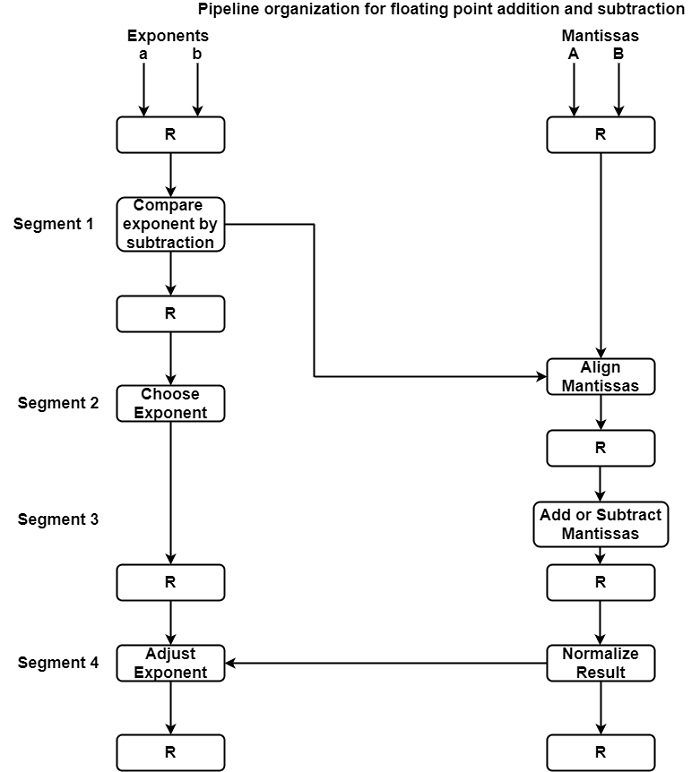# What is Arithmetic Pipeline in Computer Architecture?

Pipeline arithmetic units are generally discovered in very large-speed computers. It can execute floating-point operations, multiplication of fixed-point numbers, and the same computations encountered in mathematical problems.

The inputs to the floating-point adder pipeline are two normalized floating-point binary numbers represented as −

X = A x 2a

Y = B x 2b

Where A and B are two fractions that define the mantissa and a and b are the exponents. The floating-point addition and subtraction can be implemented in four segments, as a displayed figure. The registers labeled R is located between the segments to save intermediate outcomes. The suboperations that are implemented in the four segments are −

• It can compare the exponents.
• It can align the mantissa.
• It can add or subtract the mantissa.
• It can normalize the result.

The following block diagram describes the suboperations implemented in each segment of the pipeline.## Compare exponent by Subtraction

The exponents are compared by subtracting them to regulate their difference. The higher exponent is selected as the exponent of the result. The exponent difference regulates how many times the mantissa related with the smaller exponent should be transferred to the right.

## Align the mantissa

The mantissa related to the smaller exponent is transferred as per the difference of exponents regulate in segment one.

X = 0.9504 * 103

Y = 0.08200 * 103